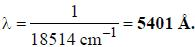Courses

# Test: Atomic & Molecular Physics - 1

## 20 Questions MCQ Test GATE Physics Mock Test Series | Test: Atomic & Molecular Physics - 1

Description
This mock test of Test: Atomic & Molecular Physics - 1 for GATE helps you for every GATE entrance exam. This contains 20 Multiple Choice Questions for GATE Test: Atomic & Molecular Physics - 1 (mcq) to study with solutions a complete question bank. The solved questions answers in this Test: Atomic & Molecular Physics - 1 quiz give you a good mix of easy questions and tough questions. GATE students definitely take this Test: Atomic & Molecular Physics - 1 exercise for a better result in the exam. You can find other Test: Atomic & Molecular Physics - 1 extra questions, long questions & short questions for GATE on EduRev as well by searching above.
QUESTION: 1

### The radius of the first Bohr orbit in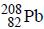for a μ-mesic (or muonic) atom is ( The masses of μ– -meson and proton are 207 times and 1836 times respectively the mass of electron).

Solution:

The radius of the first orbit (n = 1) of a hydrogen like muonic atomtaking finite mass ofnucleus in consideration is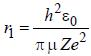....(i) where μ is reduced mass.
The reduced mass of the muonic atom (formed by a μ-meson and a proton) is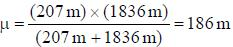, where m is the mass o f the electron.
Thus. equation (i) ⇒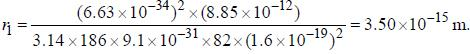QUESTION: 2

### The moment of inertia of the HCl molecule is 2.71 x 10-47 kg-m2. The most populated rotational level for the molecule at temperature of 600 K corresponds to

Solution:

The value of rotational quantum number J tor the maximum populated rotational level for a molecule at a temperature T is given by J =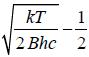, where B is rotational constant.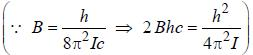∴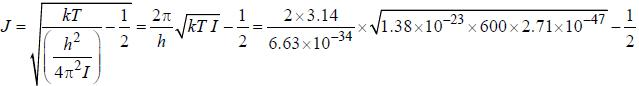= 4.5 - 0.5 =4.

QUESTION: 3

### The magnetic moment of an election moving in a circular orbit of radius r about a proton is where =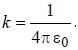Solution:

The magnetic moment is given by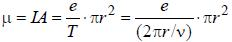...(i)
For electron the required centripetal force comes from Coulombic attraction between nucleus and electron.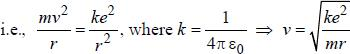...(ii)
From equation (ii), equation (i) ⇒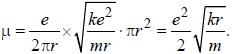QUESTION: 4

The normal Zeeman splitting in the mercury 4916 Å line in a magnetic field of 0.3 T is

Solution:

The Zeeman splitting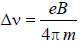....(i)
∵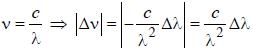∴ Equation (i) ⇒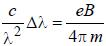⇒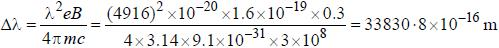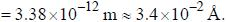QUESTION: 5

The common wave number difference in the two successive rotational lines is

Solution:

Tlie commom wave number difference in the two successive rotational lives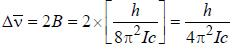QUESTION: 6

For high principal quantum number (n) for hydrogen atom the spacing between the neighbouring energy level is proportional to

Solution:

The difference of energy between energy levels having principal quantum number n and (n + 1) is given by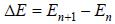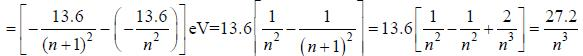*Answer can only contain numeric values
QUESTION: 7

The Lande g-factor for the 3P2 level of an atom is______________
(Round off to one decimal places)

Solution:

The Lande’s g-factor is defined as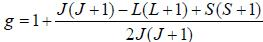...(i)
For 3P2, S = 1, L = 1, J = 2
Therefore, equation (i) ⇒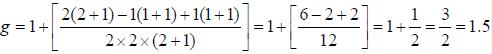*Answer can only contain numeric values
QUESTION: 8

The Bohr model gives the value for the ionization potential of Be+++ ion as__________10-1 eV
(Round off to two decimal places)

Solution:

The ionization energy of Be+++ = Z2 x ionization energy of hydrogen atom
= (4)2x 13.6 e V = 16 x 13.6 eV = 217.6 eV.

*Answer can only contain numeric values
QUESTION: 9

The line width of a spectral line of 600 mil wavelength emitted from a level of a lifetime of 10-8  sec, is__________10-15 m (up to two decimal places)

Solution:

Since,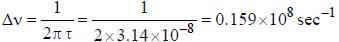For λ = 600 nm, the line width is given by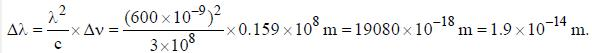*Answer can only contain numeric values
QUESTION: 10

The radiations given offby the Hg atoms returning to their normal states were studied by Frank and Heitz and
a line was observed at 2573 Å. The excitation potential for Hg is________volt (upto one decimal place)

Solution:

The excitation potential is given by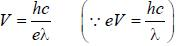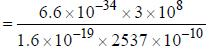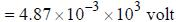= 4.9 volt.

*Answer can only contain numeric values
QUESTION: 11

The spacing of a series of lines in the microwave spectrum of AIH is constant at 12.604 cm-1. The moment of
inertia of the AIH molecule is_______x 10-47 kg/m2. (upto two decimal places)

Solution:

Since,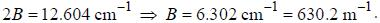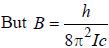, where I is the moment of inertia of the rotating molecule.
Therefore,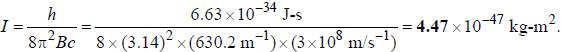*Answer can only contain numeric values
QUESTION: 12

If the Kα -radiation o f Mo (Z = 42) lias a wavelength o f 0.71 A. the wavelength o f the corresponding radiation for Cu (Z = 29) is Å. (upto two decimal places)

Solution:

From Moseley's law to r Kα - line, we have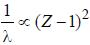Therefore,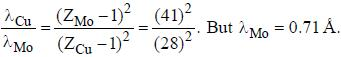Therefore,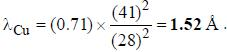QUESTION: 13

The possible values of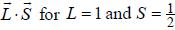is

Solution:

The total angular momentum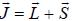Taking self dot product on both sides, we get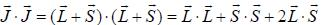⇒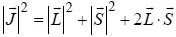⇒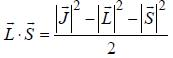⇒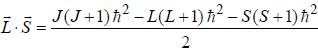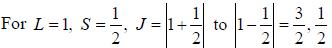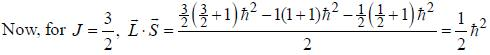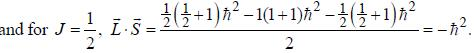QUESTION: 14

A positroniiun atom is system consisting of a position and an electron revolving about their common center of mass, which lies halfway between them. The ratio of the wavelength of the first line of the Balmer series of positroniumatomto the wavelength of the first line of the Balmer seiies of hydrogen atom is

Solution:

For posittronium atom reduced mass,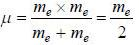The Rydbery constant for positronium atom, RP is given by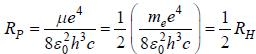...(1)
Now, the wavelength of Balmer first line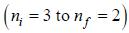of positronium a tom is given by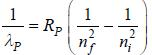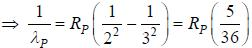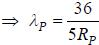....(2)
Similarly, for hydrogen atom, the wavelength of Balmer first line (ni = 3 to nf = 2) is given by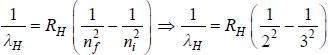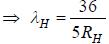Now, the ratio of wavelengths of first line o f Balmer series o fpositronium a tom and hydrogen a tom is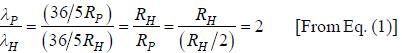QUESTION: 15

A sample of ordinary hydrogen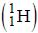gas in a discharge tube was seen to emit the usual Balmer spectrum. On careful examination however, it was found that the Hα -line in the spectrum was split into two fine lines, one an intense line at 6562.80 Å and the other at 6561.01 Å. From this, one can conclude that the gas sample had a small impurity of

Solution: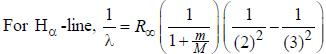⇒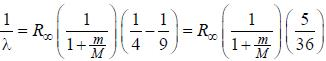⇒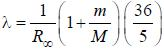.....(i)
For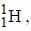the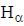-line is at 6562.80 Å. thus equation (i) implies with M = 1836 m, λ = 6562.80 Å;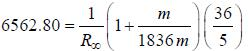...(ii)
For unknown impurity, the- line is at 6561.01 Å thus M = x x 1836 m (H ere, we have assum ed the m ass of nucleus of unknown impurity equal to x times mass of proton or hydrogen nucleus). λ = 6561.01 Å;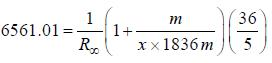...(iii)
Now. dividing equation (ii) by (iii), we get 1.000272824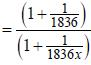⇒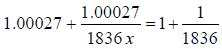⇒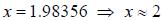Hence, the mass o f nucleus is 2 times that of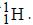Out o f the given options, nucleus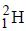has the mass equal to two times the nuclear mass of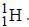QUESTION: 16

The gBe+ ion has a nucleus with spin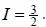The possible values of the hypofine quantum number F for the 2S1/2 electric level are

Solution:

For 2S1/2 electronic level: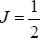For gBe+ ion, I = 3/2
The hyperfine quantum number F is defined as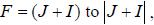with decrease o f unity.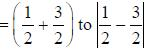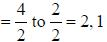*Answer can only contain numeric values
QUESTION: 17

An atomic magnetic dipole having orbital magnetic moment of 1 Bohr magneton is aligned parallel to a external magnetic field of 1 Tesla. The energy required to turn the dipole to align it antiparallel to the field is_________x 10-4 eV. (upto two decimal places)

Solution:

The potential energy of dipole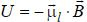For parallel alignment, θ = 0°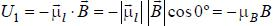...(i)
For antiparallel alignment, θ = 180°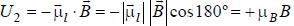...(ii)
The energy required to align the dipole in antiparallel direction to the field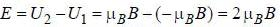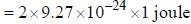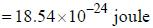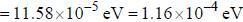QUESTION: 18

In the doublet splitting of the first excited state. 22P3/2 — 2 2P1/2 of He+ is 5.84 cm-1. the corresponding separation for H is

Solution:

Since, doublet splitting of a one election atomic state arising due to spin orbit interaction is given by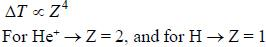Therefore,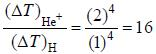Therefore,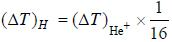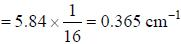QUESTION: 19

Assume that the H2 molecule behaves like a harmonic oscillator with a force constant k = 573 N/m the vibrational quantum number, corresponding to its dissociation energy 4.5 eV, is

Solution:

For H2, the reduced mass (μ)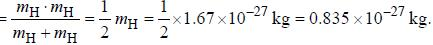Therefore, frequency of oscillation of the molecule is given by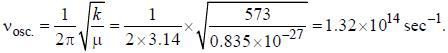For vibrational quantum number (n) corresponding to dissociation energy (Ediss), we have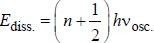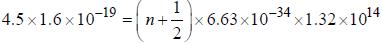⇒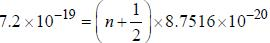⇒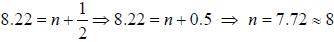*Answer can only contain numeric values
QUESTION: 20

If the exciting line in an experiment is 5460 Å and the stokes line is at 5520 Å. the wavelength (inÅ) of the antistokes line is__________Å. (should be an integer)

Solution:

Since, the stokes and the anti-stokes lines have the same wave number difference with respect to the exciting line. The w ave num ber o f the exciting line is v =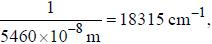and that of the stokes line is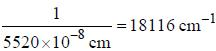Thus, the wave num ber displacement is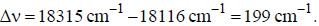Therefore, the wave number corresponding to the antistokes line would be given by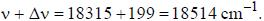The corresponding wavelength is